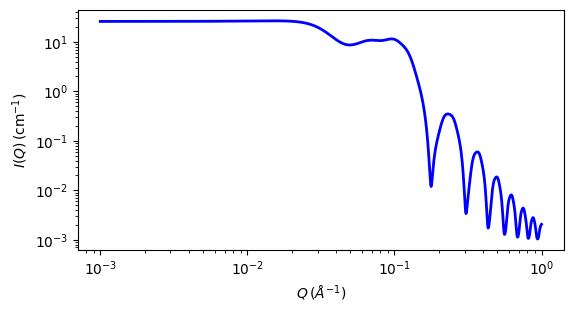# binary_hard_sphere

binary mixture of hard spheres with hard sphere interactions.

Parameter

Description

Units

Default value

scale

Scale factor or Volume fraction

None

1

background

Source background

cm-1

0.001

radius of large particle

100

radius of small particle

25

volfraction_lg

volume fraction of large particle

None

0.1

volfraction_sm

volume fraction of small particle

None

0.2

sld_lg

scattering length density of large particle

10-6-2

3.5

sld_sm

scattering length density of small particle

10-6-2

0.5

sld_solvent

Solvent scattering length density

10-6-2

6.36

The returned value is scaled to units of cm-1 sr-1, absolute scale.

Definition

The binary hard sphere model provides the scattering intensity, for binary mixture of hard spheres including hard sphere interaction between those particles, using rhw Percus-Yevick closure. The calculation is an exact multi-component solution that properly accounts for the 3 partial structure factors as follows:

$I(q) = (1-x)f_1^2(q) S_{11}(q) + 2[x(1-x)]^{1/2} f_1(q)f_2(q)S_{12}(q) + x\,f_2^2(q)S_{22}(q)$

where $$S_{ij}$$ are the partial structure factors and $$f_i$$ are the scattering amplitudes of the particles. The subscript 1 is for the smaller particle and 2 is for the larger. The number fraction of the larger particle, ($$x = n2/(n1+n2)$$, where $$n$$ = the number density) is internally calculated based on the diameter ratio and the volume fractions.

\begin{align*} x &= \frac{(\phi_2 / \phi)\alpha^3}{(1-(\phi_2/\phi) + (\phi_2/\phi) \alpha^3)} \\ \phi &= \phi_1 + \phi_2 = \text{total volume fraction} \\ \alpha &= R_1/R_2 = \text{size ratio} \end{align*}

The 2D scattering intensity is the same as 1D, regardless of the orientation of the q vector which is defined as

$q = \sqrt{q_x^2 + q_y^2}$

NOTE 1: The volume fractions and the scattering contrasts are loosely correlated, so holding as many parameters fixed to known values during fitting will improve the robustness of the fit.

NOTE 2: Since the calculation uses the Percus-Yevick closure, all of the limitations of that closure relation apply here. Specifically, one should be wary of results for (total) volume fractions greater than approximately 40%. Depending on the size ratios or number fractions, the limit on total volume fraction may be lower.

NOTE 3: The heavy arithmetic operations also mean that at present the function is poorly behaved at very low qr. In some cases very large qr may also be poorly behaved. These should however be outside any useful region of qr.

The code for this model is based originally on a c-library implementation by the NIST Center for Neutron Research (Kline, 2006).

See the references for details.Fig. 76 1D plot corresponding to the default parameters of the model.

Source

binary_hard_sphere.py $$\ \star\$$ binary_hard_sphere.c $$\ \star\$$ sas_3j1x_x.c

References

1. N W Ashcroft and D C Langreth, Physical Review, 156 (1967) 685-692 [Errata found in Phys. Rev. 166 (1968) 934]

2. S R Kline, J Appl. Cryst., 39 (2006) 895

Authorship and Verification

• Author: NIST IGOR/DANSE Date: pre 2010

• Last Modified by: Paul Butler Date: March 20, 2016

• Last Reviewed by: Paul Butler Date: March 20, 2016